### The SCAN Method

The smallest canonical (SCAN) correlation method can tentatively identify the orders of a stationary or nonstationary ARMA process. Tsay and Tiao (1985) proposed the technique, and Box, Jenkins, and Reinsel (1994) and Choi (1992) provide useful descriptions of the algorithm.

Given a stationary or nonstationary time series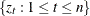with mean corrected form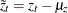with a true autoregressive order of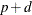and with a true moving-average order of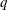, you can use the SCAN method to analyze eigenvalues of the correlation matrix of the ARMA process. The following paragraphs provide a brief description of the algorithm.

For autoregressive test order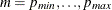and for moving-average test order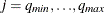, perform the following steps.

1. Let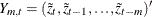. Compute the following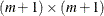matrix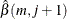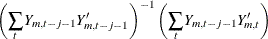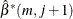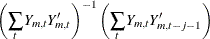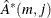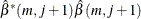whereranges from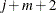to.

2. Find the smallest eigenvalue,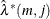, of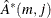and its corresponding normalized eigenvector,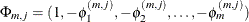. The squared canonical correlation estimate is.

3. Using the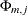as AR() coefficients, obtain the residuals for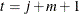to, by following the formula: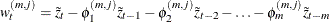.

4. From the sample autocorrelations of the residuals,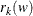, approximate the standard error of the squared canonical correlation estimate by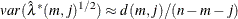where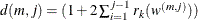.

The test statistic to be used as an identification criterion is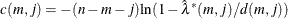which is asymptotically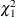if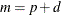and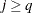or if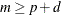and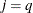. For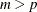and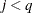, there is more than one theoretical zero canonical correlation between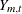and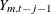. Since theare the smallest canonical correlations for each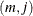, the percentiles of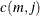are less than those of a; therefore, Tsay and Tiao (1985) state that it is safe to assume a. For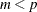and, no conclusions about the distribution ofare made.

A SCAN table is then constructed usingto determine which of theare significantly different from zero (see Table 7.7). The ARMA orders are tentatively identified by finding a (maximal) rectangular pattern in which theare insignificant for all test orders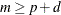and. There may be more than one pair of values (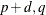) that permit such a rectangular pattern. In this case, parsimony and the number of insignificant items in the rectangular pattern should help determine the model order. Table 7.8 depicts the theoretical pattern associated with an ARMA(2,2) series.

Table 7.7: SCAN Table

 MA AR 0 1 2 30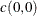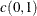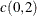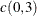1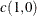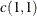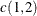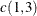2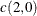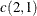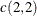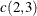3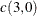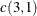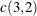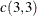Table 7.8: Theoretical SCAN Table for an ARMA(2,2) Series

 MA AR 0 1 2 3 4 5 6 7 0 * X X X X X X X 1 * X X X X X X X 2 * X 0 0 0 0 0 0 3 * X 0 0 0 0 0 0 4 * X 0 0 0 0 0 0 X = significant terms 0 = insignificant terms * = no pattern